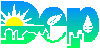Water/Wastewater Math Calculator
Temperature Calculator

Formula:

C° x 1.8 + 32
= F°

***

F° - 32 / 1.8
= C°

Temperature
Convert Celsius to Fahrenheit

 Water/Wastewater math calculator. Select the calculator you want, then press GO! Select your Calculator - then press GO! Tank (or clarifier) Volume Calculator Removal Efficiency (wastewater) Loadings Calculator (Lbs) F/M (activated Sludge) Calculator